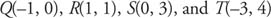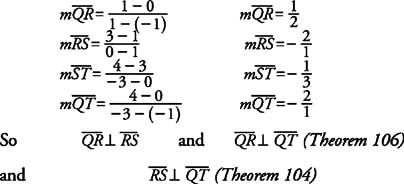## Slopes: Parallel and Perpendicular Lines

If lines are parallel, they slant in exactly the same direction. If they are nonvertical, their steepness is exactly the same.

Theorem 103: If two nonvertical lines are parallel, then they have the same slope.

Theorem 104: If two lines have the same slope, then the lines are nonvertical parallel lines.

If two lines are perpendicular and neither one is vertical, then one of the lines has a positive slope, and the other has a negative slope. Also, the absolute values of their slopes are reciprocals.

Theorem 105: If two nonvertical lines are perpendicular, then their slopes are opposite reciprocals of one another, or the product of their slopes is −1.

Theorem 106: If the slopes of two lines are opposite reciprocals of one another, or the product of their slopes is −1, then the lines are nonvertical perpendicular lines.

Horizontal and vertical lines are always perpendicular: therefore, two lines, one of which has a zero slope and the other an undefined slope are perpendicular.

Example 1: If line l has slope 3/4, then (a) any line parallel to l has slope ___, and (b) any line perpendicular to l has slope ___.

a. (a) 3/4 (Theorem 103)

b. (b) −4/3 (Theorem 105)

Example 2: Given points Q, R, S, and T, tell which sides, if any, of quadrilateral QRST in Figure 1 are parallel or perpendicular.Figure 1 Determining which sides, if any, of a quadrilateral are parallel or perpendicular.Back to Top
A18ACD436D5A3997E3DA2573E3FD792A